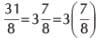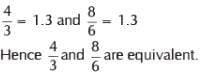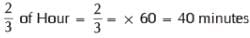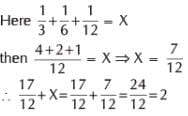# Olympiad Test: Fractions - 1

Test Description

## 10 Questions MCQ Test Mathematics Olympiad Class 4 | Olympiad Test: Fractions - 1

Olympiad Test: Fractions - 1 for Class 4 2023 is part of Mathematics Olympiad Class 4 preparation. The Olympiad Test: Fractions - 1 questions and answers have been prepared according to the Class 4 exam syllabus.The Olympiad Test: Fractions - 1 MCQs are made for Class 4 2023 Exam. Find important definitions, questions, notes, meanings, examples, exercises, MCQs and online tests for Olympiad Test: Fractions - 1 below.
 1 Crore+ students have signed up on EduRev. Have you?
Olympiad Test: Fractions - 1 - Question 1

### Pick the odd one out.

Detailed Solution for Olympiad Test: Fractions - 1 - Question 1

21/5 is not a proper fraction.

Olympiad Test: Fractions - 1 - Question 2

### Write 31/8 as a mixed number.

Detailed Solution for Olympiad Test: Fractions - 1 - Question 2Olympiad Test: Fractions - 1 - Question 3

### A fraction a/b = 1, when

Detailed Solution for Olympiad Test: Fractions - 1 - Question 3

A/B = 1 is possible when A = B

Olympiad Test: Fractions - 1 - Question 4

Express 400 ml as a fraction of 1.

Olympiad Test: Fractions - 1 - Question 5

Pick the odd one out.

Olympiad Test: Fractions - 1 - Question 6

Which two fractions are equivalent?

Detailed Solution for Olympiad Test: Fractions - 1 - Question 6Olympiad Test: Fractions - 1 - Question 7

Evaluate 5 (2/3) – 3 (1/2) =

Olympiad Test: Fractions - 1 - Question 8

How many minutes are there in 2/3 of an hour?

Detailed Solution for Olympiad Test: Fractions - 1 - Question 8Olympiad Test: Fractions - 1 - Question 9

If 1/3 + 1/6 + 1/12 = X, then X + 17/12 = ?

Detailed Solution for Olympiad Test: Fractions - 1 - Question 9Olympiad Test: Fractions - 1 - Question 10

Chose the incorrect option of the following :